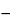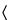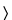Calcium Ascorbate
(kal' see um a skor' bate).

C12H14CaO12·2H2O 426.34
DEFINITION
Calcium Ascorbate contains NLT 98.0% and NMT 101.0% of calcium ascorbate dihydrate (C12H14CaO12·2H2O), calculated on the as-is basis.
IDENTIFICATION
A 100 mg/mL solution meets the requirements.
•  B. A 100 mg/mL solution decolorizes a 100 mg/mL solution of dichlorophenol–indophenol.
ASSAY
•  Procedure
Sample:  300 mg of Calcium Ascorbate
Blank:  50 mL of water
Titrimetric system
Mode:  Direct titration
Titrant:  0.1 N iodine VS
Endpoint detection:  Visual
Analysis:  Transfer the Sample into a 250-mL conical flask, add 50 mL of water, and mix to dissolve. Immediately titrate with the Titrant, adding 3 mL of starch TS as the endpoint is approached. Perform a Blank determination.
Calculate the percentage of calcium ascorbate dihydrate (C12H14CaO12·2H2O) in the Sample taken:
Result = {[(VSVB) × N × F]/W} × 100
 VS = = Titrant volume consumed by the Sample (mL) VB = = Titrant volume consumed by the Blank (mL) N = = Titrant normality (mEq/mL) F = = equivalency factor, 106.6 mg/mEq W = = Sample weight (mg)
Acceptance criteria:  98.0%–101.0% on the as-is basis
IMPURITIES
•  Limit of Fluoride
[Note—Prepare and store all solutions in plastic containers. ]
Buffer solution:  294 mg/mL of sodium citrate dihydrate in water
Standard stock solution:  1.1052 mg/mL of USP Sodium Fluoride RS in water
Standard solution:  Transfer 20.0 mL of Standard stock solution to a 100-mL volumetric flask containing 50.0 mL of Buffer solution, dilute with water to volume, and mix. Each mL of Standard solution contains 100 µg of fluoride ion.
Sample solution:  Transfer 2.0 g of Calcium Ascorbate to a beaker containing a plastic-coated stirring bar. Add 20 mL of water and 2.0 mL of hydrochloric acid, and stir until dissolved. Add 50.0 mL of Buffer solution and sufficient water to make 100 mL.
Electrode system:  Use a fluoride-specific ion-indicating electrode and a silver–silver chloride reference electrode connected to a pH meter capable of measuring potentials with a minimum reproducibility of ± 0.2 mV (see pH791).
Analysis
Samples:  Standard solution and Sample solution
Standard response line:  Transfer 50.0 mL of Buffer solution and 2.0 mL of hydrochloric acid to a beaker, and add water to make 100 mL. Add a plastic-coated stirring bar, insert the electrodes into the solution, stir for 15 min, and read the potential, in mV. Continue stirring, and at 5-min intervals add 100, 100, 300, and 500 µL of Standard solution, reading the potential 5 min after each addition. Plot the logarithms of the cumulative fluoride ion concentrations (0.1, 0.2, 0.5, and 1.0 µg/mL) versus potential, in mV.
Rinse and dry the electrodes, insert them into the Sample solution, stir for 5 min, and read the potential, in mV. From the measured potential and the Standard response line determine the concentration, C (in µg/mL), of fluoride ion in the Sample solution.
Calculate the content of fluoride in the portion of Calcium Ascorbate taken:
Result = (C × V)/W
 C = = concentration of fluoride ion in the Sample solution (µg/mL), obtained from the Standard response line V = = volume of the Sample solution (mL) W = = weight of Calcium Ascorbate taken to prepare the Sample solution (g)
Acceptance criteria:  NMT 10 ppm
SPECIFIC TESTS
Sample solution:  50 mg/mL in carbon dioxide-free water [Note—Perform measurements immediately after preparation. ]
Acceptance criteria:  +95to +97Sample solution:  100 mg/mL
Acceptance criteria:  6.8–7.4
Dry 3 g at 105for 2 h: it loses NMT 0.1% of its weight.